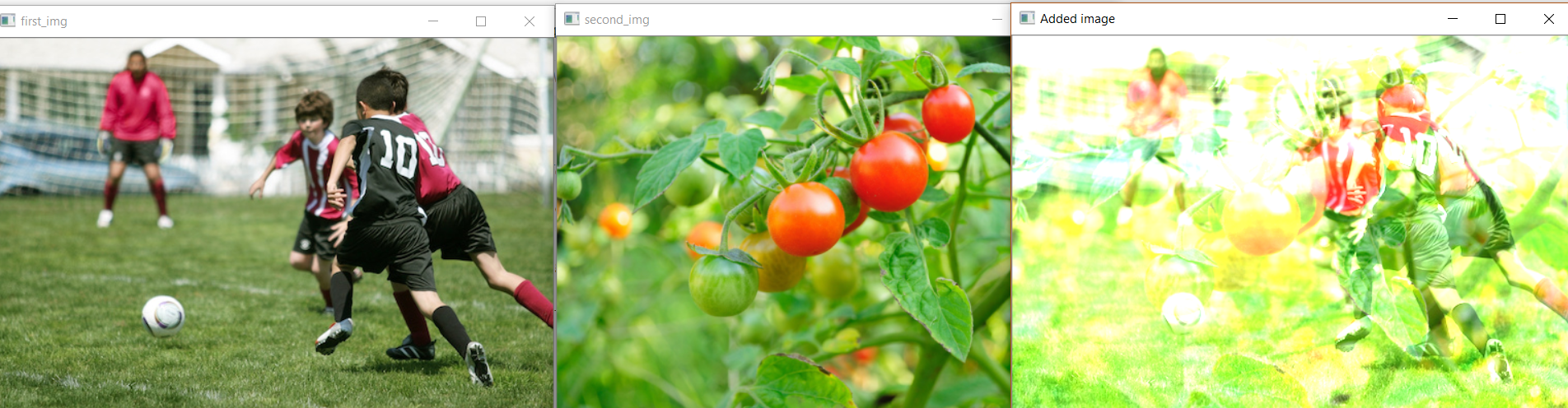# Arithmetic operations using OpenCV | Python

We can perform different Arithmetic operations on images e.g. Addition, Subtraction, etc. This is possible because images are actually stored as arrays (3 Dimensional for RGB images and 1 dimensional for the grayscale images).

Importance of Arithmetic Operations on images:

• Image Blending: Addition of Images is used for image blending where images are multiplied with different weights and added together to give a blending effect.
• WaterMarking: It is also based on the principle of addition of very low weight image addition to the original image.
• Detecting changes in image: Image subtraction can help in identifying the changes in two images as well as to level uneven sections of the image e.g. to handle half part of image which has shadow on it.

 `import`  `cv2 ` `import` `matplotlib.pyplot as plt ``%` `matplotlib inline ` `# matplotlib can be used to plot the images as subplot ` ` `  `first_img ``=` `cv2.imread(``"C://gfg//image_processing//players.jpg"``) ` `second_img ``=` `cv2.imread(``"C://gfg//image_processing//tomatoes.jpg"``) ` ` `  `print``(first_img.shape) ` `print``(second_img.shape) ` ` `  `# we need to resize, as they differ in shape ` `dim ``=``(``544``, ``363``) ` `resized_second_img ``=` `cv2.resize(second_img, dim, interpolation ``=` `cv2.INTER_AREA) ` `print``(``"shape after resizing"``, resized_second_img.shape) ` ` `  `added_img ``=` `cv2.add(first_img, resized_second_img) ` ` `  `cv2.imshow(``"first_img"``, first_img) ` `cv2.waitKey(``0``) ` `cv2.imshow(``"second_img"``, resized_second_img) ` `cv2.waitKey(``0``) ` `cv2.imshow(``"Added image"``, added_img) ` `cv2.waitKey(``0``) ` ` `  `cv2.destroyAllWindows() `

Output:
(363, 544, 3)
(500, 753, 3)
shape after resizing (363, 544, 3)Code for image Subtraction –

 `import`  `cv2 ` `import` `matplotlib.pyplot as plt ``%` `matplotlib inline ` ` `  ` `  `first_img ``=` `cv2.imread(``"C://gfg//image_processing//players.jpg"``) ` `second_img ``=` `cv2.imread(``"C://gfg//image_processing//tomatoes.jpg"``) ` ` `  `print``(first_img.shape) ` `print``(second_img.shape) ` ` `  `# we need to resize, as they differ in shape ` `dim ``=``(``544``, ``363``) ` `resized_second_img ``=` `cv2.resize(second_img, dim, interpolation ``=` `cv2.INTER_AREA) ` `print``(``"shape after resizing"``, resized_second_img.shape) ` ` `  `subtracted ``=` `cv2.subtract(first_img, resized_second_img) ` `cv2.imshow(``"first_img"``, first_img) ` `cv2.waitKey(``0``) ` `cv2.imshow(``"second_img"``, resized_second_img) ` `cv2.waitKey(``0``) ` `cv2.imshow(``"subtracted image"``, subtracted) ` `cv2.waitKey(``0``) ` ` `  `cv2.destroyAllWindows() `

Output:
(363, 544, 3)
(500, 753, 3)
shape after resizing (363, 544, 3)My Personal Notes arrow_drop_upCheck out this Author's contributed articles.

If you like GeeksforGeeks and would like to contribute, you can also write an article using contribute.geeksforgeeks.org or mail your article to contribute@geeksforgeeks.org. See your article appearing on the GeeksforGeeks main page and help other Geeks.

Please Improve this article if you find anything incorrect by clicking on the "Improve Article" button below.

Article Tags :

Be the First to upvote.

Please write to us at contribute@geeksforgeeks.org to report any issue with the above content.